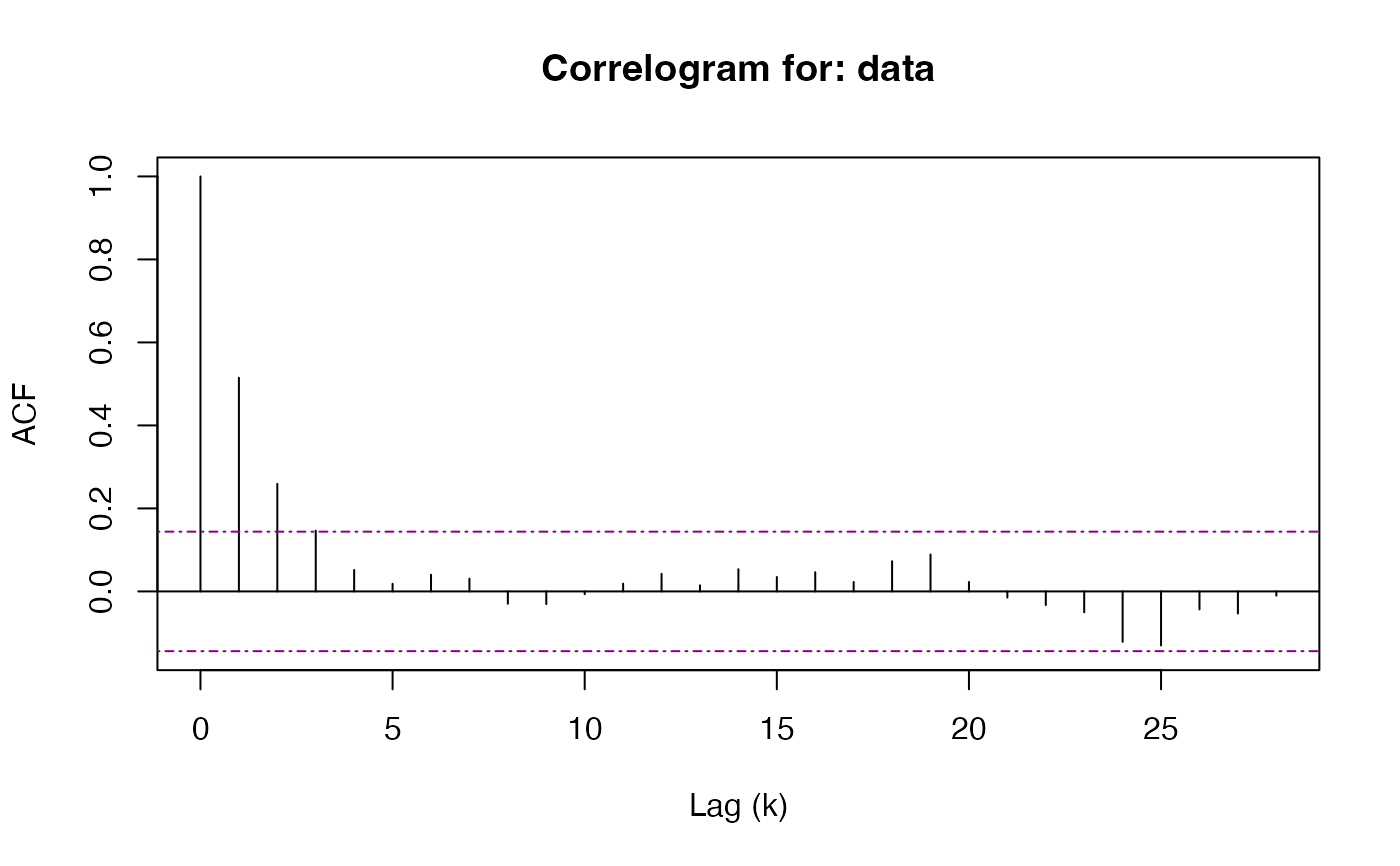S3 Method for objects of class 'pwsd' See ?plot.acf of the stats package for more customization options on the correlogram, from which plot.pwsd is based

# S3 method for pwsd
plot(x, c = NULL, main = NULL, ylim = NULL, ...)

## Arguments

x an of object of class 'pwsd' or 'acf' a numeric value, the constant which acts as the significance level for the implied hypothesis test. Defaults to qnorm(0.975) for a two-tailed 95% confidence level. Politis and White (2004) suggest c = 2. an overall title for the plot, if no string is supplied a default title will be populated. See title a numeric of length 2 giving the y-axis limits for the plot Arguments passed on to base::plot ythe y coordinates of points in the plot, optional if x is an appropriate structure.

## Value

No return value, called for side effects

## Examples

# Use S3 Method

# Generate AR(1) time series
sim <- stats::arima.sim(list(order = c(1, 0, 0), ar = 0.5),
n = 500, innov = rnorm(500))

b <- pwsd(sim, round = TRUE, correlogram = FALSE)
plot(b)